• 文章目录通用形式简介重要概念指数族分布线性回归简介正则基扩展和核函数对数几率回归简介多分类策略凸函数求解一些问题# 参考链接 通用形式 简介 ​ 广义线性模型（Generalized Linear Model），将线性模型...
文章目录通用形式简介重要概念指数族分布线性回归简介正则化基扩展和核函数对数几率回归简介多分类策略凸函数求解一些问题# 参考链接

通用形式
简介
​		广义线性模型（Generalized Linear Model），将线性模型通过联结函数（link function）联结线性预测值（linear prediction）（即线性模型的直接输出）和期望输出值（expected value）.；通过寻找因变量（dependent variables）所属的指数族分布（exponential  family distribution），建立合适联结函数，将线性模型的输出映射到期望输出，损失函数通常是指数族分布的似然函数（likelihood function）..；即采用极大似然函数求取损失函数。
\begin{aligned} LM:\; &{\hat{y}}=W^\top X+\epsilon\\ GLM:\; &{\hat{y}}=g(W^\top X+\epsilon)\\ \end{aligned}
​		可采用一对一（One Versus One）、一对多（One Versus Rest）、归一化指数函数（softmax function）等策略转变为多分类问题.。
$softmax:\;S_i=\frac{\exp{z_i}}{\sum_{j=1}^n\exp{z_j}}$
​		广义线性模型由于使用了联结函数，所以对数据分布有假设（指数族分布）；所以可进行一定的预处理使数据分布更符合指数族分布，有助于提高模型得分（model score）。
​		对比极大似然估计（Maximum Likelihood Estimate, MLE）本质，可知广义线性模型和极大似然估计都有假设，且进行逐点估计（point-wise estimate），因此；
\begin{aligned} {\color{gray}{posterior}} &= {\color{red}{likelihood}} * {\color{blue}{prior}} / {\color{green}{evidence}}\\ MLE: {\color{gray}{P(\alpha∣y)}} &= {\color{red}{P(y∣\alpha)}} * {\color{blue}{P(\alpha)}} / {\color{green}{P(y)}}\\ &={\color{red}{L(\alpha∣y)}} * {\color{blue}{P(\alpha)}} / {\color{green}{P(y)}}\\ {\color{red}{L(\alpha∣y)}} &=\max_\alpha{\sum_i\ln{p(y_i|\alpha)}} \end{aligned}

重要概念
​		属性：参数模型（parametric models）、监督学习（supervised learning）、判别模型（discriminant model）、支持核方法（kernel methods）、面试常考。
​		求解：使用指数族分布的似然损失函数计算经验风险（empirical risk），可加入正则化项（normalization term）计算结构风险（structural risk）：凸函数证明后1.进行凸优化（convex optimization），2.使用梯度下降方法，包括随机梯度下降（Stochastic Gradient Descent）、牛顿法（Newton method）。
​		扩展：广义线性模型无法解决非线性模型问题，可引入核方法；线性回归正则化的三种形式；对数几率回归扩展到多元回归（softmax regression）。

指数族分布
指数族分布是指概率密度函数（probability density function）满足下面分布公式..：
\begin{aligned} &f(y;\theta,\phi)=A(\phi)\exp[\frac{y\cdot\theta-B(\theta)}{\phi}−C(y,\phi)] \\ &X\beta=g(\mu)\\ define: &\theta:自然实参;\phi:离散实参\\ &A:单参实函;B:单参实函;C:双参实函\\ &g:联结函数\\ \end{aligned}
举例：高斯分布（$x\sim N(\mu,\sigma^2)$），也是线性回归中$\epsilon$的假设分布（假设数据沿真实回归线左右呈高斯分布），故可知：设分布方差$\sigma$对线性回归参数不干扰..
\begin{aligned} 概率密度函数: f_{g}(x;\mu,\sigma^2) &=\frac{1}{\sigma\sqrt{2\pi}}\exp(-\frac{(x-\mu)^2}{2\sigma^2})\\ 指数族分布： f(y;{\color{blue}{\theta}},{\color{orange}{\phi}}) &={\color{red}{A(\phi)}} \exp[\frac{y\cdot {\color{blue}{\theta}}-{\color{green}{B(\theta)}}} {{\color{orange}{\phi}}}−{\color{brown}{C(y,\phi)}}]\\ f_{g}(x;{\color{blue}{\mu}},{\color{orange}{\sigma^2}}) &={\color{red}{\frac{1}{\sigma\sqrt{2\pi}}}} \exp[{\color{gray}{-2}}\frac{x\cdot {\color{blue}{\mu}}-{\color{green}{\frac{1}{2}\mu^2}}} {{\color{orange}{\sigma^2}}}-{\color{brown}{x^2\sigma^2}}] \\ 联结函数：g(\mu) &=X\beta=\mu \\ \end{aligned}\\

线性回归
线性回归作为最简单的广义线性模型，本身拥有以下假设：为什么线性回归中 要将自变量设定为非随机变量？伍德里奇的导论好像是设为非随机变量的，

${\hat{y}},\epsilon$ 服从指数族分布（高斯分布）
$W,X$ 有非随机性（确定性变量，分布确定）
$g(\mu)=\mu$是联结函数（恒等式）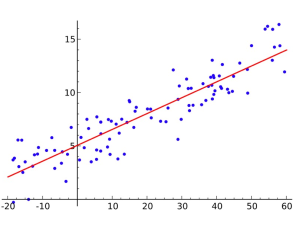简介
用回归分析拟合确定多个变量间的线性定量关系的方法，由于线性回归求解较为简单，使用解析解即可（面试可能需要最小二乘法(Least Squares Method)推导）：
\begin{aligned} LM:\; &{\hat{y}}=W^\top X+\epsilon\\ LinR:\; &{\hat{y}}=g(W^\top X+\epsilon)\\ &\;\;=W^\top X+\epsilon\\ closed\,form:\;&\hat{W}=\left( X^\top X \right)^{\color{red}{-1}}X^\top Y\\ \end{aligned}
​		根据以上解析解（closed form/analytical solution）公式，可知（暂不推导），解析解有两大约束：1.$X^\top X$可逆，2.$X$自身不应存在线性相关（此时等于共线性）；解决办法可有：1.伪逆矩阵代替不可逆矩阵（numpy.linalg.pinv()），2.去除线性相关时，线性不相关可以粗略视为：columns$\leq$rows；
​		线性回归存在2个假设：1.线性假设，即自变量和因变量成线性关系，但自变量自身互不成线性关系（共线性，collinearity）；2.分布假设，即自变量围绕最优回归拟合线两侧成高斯分布，也就是$\epsilon$应尽可能符合高斯分布假设；
​		因此实际应用中，可以使用3种方法提高拟合准确度：

样本、标签转换为线性关系： 指数关系的对数转换；

删除共线性：去除（remove），组合（combine），新算法（如PCA和PLSR） ；

样本规范化：使用变换是输入输出都近似高斯（boxcox） 并减小值大小（距离相关）影响；
$boxcox:{\hat{y}}=\begin{cases}\frac{(y+c)^\lambda-1}{\lambda} & \lambda\neq 0\\\log(y+c) & \lambda=0\end{cases}$

正则化
线性回归属于“古老模型”，当时正则化较少见，因此每个正则化模型都会赋予新名字（但本质是不变的），此处介绍一下三种正则化算法的名称和损失函数：

\begin{aligned}&L_{ridge}(\hat{\beta})=\sum^n_{i=1}(y_i-x'_i\hat{\beta})^2\,+\,\lambda\sum^m_{j=1}{\hat{\beta_j}^2}=\left\|y-X\hat{\beta}\right\|^2+\lambda\left\|\hat{\beta}\right\|^2\rightarrow\hat\beta_{ridge}=(X'X+\lambda I)^{-1}(X'Y) \\&L_{LASSO}=\sum^n_{i=1}(y_i-x'_i\hat{\beta})^2\,+\,\lambda\sum^m_{j=1}{\left|\hat{\beta_j}\right|}=\left\|y-X\hat{\beta}\right\|^2+\lambda\left|\hat{\beta}\right|\rightarrow\begin{cases} coordinate\;descent\\ Least\;Angle\;Regression\\\end{cases}\\&L_{enet}(\hat\beta)=\frac{\sum^n_{i=1}{(y_i-x'_i\hat{\beta})^2}}{2n}+\lambda\left(\frac{1-\alpha}{2}\sum^m_{j=1}{\hat{\beta_j}^2}\,+\,\alpha\sum^m_{j=1}{\left|\hat{\beta_j}\right|}\right),\;\begin{cases}ridge & \alpha=0\\LASSO & \alpha=1\end{cases}\end{aligned}

基扩展和核函数

由于需要扩展非线性线性回归，这里把上面不进行特殊处理的线性回归，称为简单线性回归

​		简单线性回归需要自变量和因变量成线性关系，约束了可解决问题范畴，针对此局限，可以采用基扩展（basis expansion）和核函数（kernel function），将特征$X$转为更高次特征（基扩展）和映射到高维空间（核函数），而引入非线性特征
基扩展（basis expansion）
\begin{aligned} &\qquad&Y=W^\top\phi(x)+\epsilon\\ &nomal:&\phi(x)={\color{gray}{[x]}}\\ &polyno:&\phi(x)={\color{red}{[1,x,x^2]}}\\ \end{aligned}

引入特征交叉（feature crossing），将特征转为更高次特征（非线性特征），直观理解就是提高假设空间的导数，获取更复杂模型，因此也容易过拟合，而正则化仍起作用（较小，因为导数公式常数项并未改变）
生成多项式回归（polynomial regression），即用通过高次特征，生成多项式表达式（导数更高阶），而生成复杂模型

核函数（kernel function）
KaTeX parse error: No such environment: align at position 8:
\begin{̲a̲l̲i̲g̲n̲}̲
primal:{\hat{y…
（此处使用带正则罚项的原问题形式）
​		由上式（RBF kernel）可看出，在此时（linear regression），核函数和基扩展有相似之处：公式（$W^\top\phi(x)+\epsilon$）和效果（升维），但本质（线性变换和核函数条件）不一样

对数几率回归
对数几率回归（Logistics Regression，又称逻辑回归）本身拥有以下假设：

${\hat{y}},\epsilon$ 服从指数族分布（二项分布，伯努利分布）

$W,X$ 有非随机性（确定性变量，分布确定）

$g(\mu)=\frac{1}{1+\exp(-\mu)}$是联结函数（Sigmoid函数）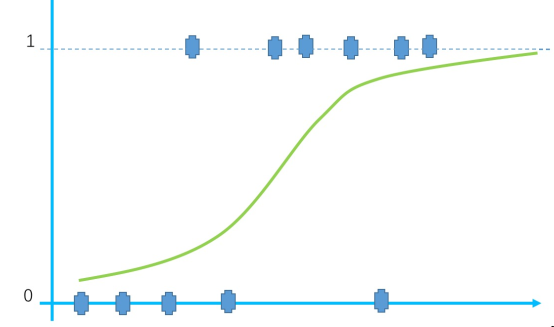简介
\begin{aligned} LM:\; &{\hat{y}}=W^\top X+\epsilon\\ LR:\; &{\hat{y}}=g(W^\top X+\epsilon)\\ &\;\;=\frac{1}{1+exp(-[W^\top X+\epsilon])}\\ \end{aligned}
​		（目前没有对数几率回归的解析解）根据通用形式-简介中最大似然估计的本质，可以简略推导损失函数（$\epsilon$ 假设服从高斯分布（均值为0），书写可以忽略 ）
\begin{aligned} LR:\;{\hat{y}} &=g(W^\top X+\epsilon)\\ &=\frac{1}{1+exp(-[W^\top X+\epsilon])}\\ MLE:\;L(\alpha|y) &=\max_\alpha{\sum_i\ln{p(y_i|\alpha)}}\\ &=\max_\alpha{\sum_i\ln{y^i\ln{p}+(1-y^i)\ln{1-p}}}\\ loss:\;J(W|{\hat{y}}) &=L(W,b|{\hat{y}})\\ &=-\min_{W,b}[{\sum_i{{\hat{y}}^i\ln{p}+ (1-{\hat{y}}^i)\ln{1-p}}}]\\ \end{aligned}
​		对数几率回归中的“几率”（odds），应用于可解释性，即解释模型参数和特征的关系，特征每变化一，新旧“对数几率”（log odds or logits）也对应变化：
\begin{aligned} odds:\;\frac{p}{1-p} &=\frac{\hat{y}}{1-\hat{y}}\\ &=\frac{1+exp(-f_{w,b}(x))}{1+exp(f_{w,b}(x))}\\ &=\exp(f_{w,b}(x))=\exp(W^\top X)\\ \log odds: &\log\frac{p}{1-p}=W^\top X \rightarrow X=\frac{\log odds}{W}\\ &\frac{X_a+1}{X_a}=\frac{X_b}{X_a}= \frac{\log odds_b}{W_b}/\frac{\log odds_a}{W_a}\\ \log adds\,ratio: &\frac{\log odds_b}{\log odds_a}= {\color{red}{\frac{W_b}{W_a}}}\cdot\frac{X_b}{X_a}\\ {\color{gray}{define:\;{\hat{y}}}} &{\color{gray}{=\frac{1}{1+exp(f_{w,b}(x))}}}\\ {\color{gray}{\;1-{\hat{y}}}} &{\color{gray}{=\frac{1}{1+exp(-f_{w,b}(x))}}}\\ \end{aligned}

多分类策略

softmax logistic regression: multi-calss, single label of sample
multi-label logistic regression: multi-calss, multi-label of sample  （$C_k^2>k,\;when\;k>3$）

OVR（one versus rest）：k元分类问题，训练$k$个模型，每个模型二分类为某个类和非该类，预测时选择$max_i\,w_i^{\rm{T}}x\;$为$x$的类别
OVO（one versus one）：k元分类问题，训练$C_k^2$个模型，每个模型二分类为k元中二元组合，预测时选择计票次数最多的$i$为$x$的类别

\begin{aligned} softmax:\;&J(w)={\sum_{i=1}^n}{\sum_{k=1}^K}{1\{y^{(i)}=k\}{log\,\frac{exp(w^{(k)\rm{T}}x^{(i)})}{\sum_{j=1}^{K}exp(w^{(j)\rm{T}}x^{(i)})}}} \end{aligned} \\

凸函数求解
​		由上面可知损失函数，可证明了该损失函数是凸函数（后续会推出凸优化博客，届时会详细推导~），即通过梯度下降等方法可寻找全局最优解（global optimal solution）：常用的求解方法如下（此处不做详细推导）：

梯度下降（SGD）
\begin{aligned} 梯度：\arg\min [f(x+\delta)-f(x)] &\approx \arg\min f\,'(x)\cdot \delta\\ &=\arg\min_\theta \| f\,'(x)\|\cdot \|\delta\|\cdot cos\theta \\ 梯度下降：W_j^{new} &= W_j + \nabla W_j\\ {\color{gray}{\cos\pi=-1}}:\qquad &=W_j-\eta\frac{\partial J(W,b)}{\partial W_j}\\ \end{aligned}

牛顿法（泰勒展开二阶式子）
\begin{aligned} 牛顿法：\phi (x) &=f(x_k)+\nabla f(x_k)(x-x_k)\\ &\qquad\qquad +\frac{1}{2}\cdot \nabla^2f(x_k)(x-x_k)(x-x_k)^{\rm{T}}\\ \nabla \phi(x) &=0+\nabla f(x_k)+[\nabla^2f(x)(x-x_k)\cdot 1]=0\\ 牛顿法下降：x &=x_k-\frac{\nabla f(x_k)}{\nabla^2f(x_k)}\qquad {\color{gray}{if\;\nabla^2f(x_k)\;not\;Singular}}\\ &=x_k-H_{hessian}^{-1}\cdot G_{gradient}\qquad {\color{gray}{G_{gradient}<\epsilon}}\\ \end{aligned}

拟牛顿法（BFGS）（直接逼近海森矩阵$B_{k}\approx H_{hessian}$）
\begin{aligned} \Delta B_k=\frac{y_ky_k^\top}{y_k^\top s_k}- \frac{B_ks_ks_k^\top B_k}{s_k^\top B_ks_k}\\ \end{aligned}

一些问题

为什么（多元）线性回归损失函数使用最小二乘法而不是似然函数？线性回归的损失函数为什么用最小二乘不用似然函数

最小二乘法以估计值与观测值的平方和作为损失函数，在误差服从正态分布的前提下，与极大似然估计的思想在本质上是相同。我们通常认为ε服从正态分布，通过对极大似然公式推导，就是最小二乘

为什么要使用sigmoid函数作为假设？LR为什么使用sigmoid函数

因为线性回归模型的预测值为实数，而样本的类标记为（0,1），我们需要将分类任务的真实标记$y$与线性回归模型的预测值$\hat{y}$联系起来。如果选择单位阶跃函数的话，它是不连续的不可微；如果选择sigmoid函数，它是连续的，而且能够将z转化为一个接近0或1的值，同时其导数特性也方便进行极大似然估计；sigmoid是伯努利分布的指数族形式

为什么LR损失函数使用交叉熵不用均方差 ？为什么LR模型损失函数使用交叉熵不用均方差

均方误差通用公式为$\frac{(y-\hat{y})^2}{2}$，其导数为${(y-\hat{y})}\sigma\,'(x\,;\theta)x$，由于$\sigma$函数导数值在两端都极小，不利于参数$\theta,\;b$利用梯度下降更新； 而交叉熵通用公式为极大似然推导，其导数为$-[y-\sigma(x\,;\theta)]x$，导数值没有值极小点，同时该导数也反映出，预测差距大 时参数更新更快

LR通用流程是什么？逻辑回归求解

数据预处理（连续型离散化，异常值，缺失值，标准化）$\rightarrow$选择求解算法（多种梯度下降、牛顿法）$\rightarrow$选择训练停止机制（STOP_ITER、STOP_COST、STOP_GRAD）

LR优缺点是什么？4.逻辑回归优缺点

判别模型（对数据少场景也可用），形式简单但可解释性强，可获得预测概率，改进后可进行多分类任务； 但准确率不高，无法筛选特征

# 参考链接

序号
平台
作者链接
内容链接
使用范畴


西瓜书
周志华
第三章
提供GLM框架，并介绍了算法实现方法和实现时注意问题


统计学简便手册
PRACTICALLY CHEATING STATISTICS HANDBOOK
Generalized Linear Model (GLZ): An Overview
（英文）关注GLM简介，并介绍了英文名词和部分指数族分布的公式


统计之都
张缔香
从线性模型到广义线性模型（1）——模型假设篇-简介部分
（有后续）精简地介绍了GLM的模型假设问题


贪心科技
自然语言处理训练营
review-线性回归、review-逻辑回归
目前（2020年）国内最好的NLP训练营


简书
secondplayer
机器学习笔记3: 广义线性模型
（有后续）详细解释了GLM，并推导高斯分布和伯努利分布作为例子


百度百科
尚轶伦（同济大学也）
线性回归
线性回归知识框架


个人网站
Jim Forst
Multicollinearity in Regression Analysis: P D and S
参考了共线性的定义和在回归中的检测方法、解决方法


ncss
unkonwn
Box-Cox Transformation for Sim Lin Reg
参考了boxcox公式以及在简单线性回归怎么使用（how to run）


TDS
Animesh Agarwal
Polynomial Regression
(1.7k likes)参考了多项式回归的应用，复习了偏差方差均衡


TDS
Jonathan Balaban
A Gentle Introduction to M L E
(2.4k likes)参考了最大似然估计的本质和线性回归中的应用


S
皮果提
牛顿法与拟牛顿法学习笔记（四）BFGS
（260点赞）基本采纳了BFGS的定义和方程组


purdue
unknown
BFGS method
（ppt）公式推导较详尽


展开全文机器学习 逻辑回归
• 闭式解也被称为解析解，知是通过严格的公式所求得的解，即包含分式、三角函数、指数、对数甚至无限级数等基本函数的解的形式。通过给出解的具体函数形式，从解的表达式中就可以算出任何对应值。 2、正则 P.S:推荐...
1、闭式解
闭式解也被称为解析解，知是通过严格的公式所求得的解，即包含分式、三角函数、指数、对数甚至无限级数等基本函数的解的形式。通过给出解的具体函数形式，从解的表达式中就可以算出任何对应值。
2、正则化
P.S:推荐参考资料 https://www.jianshu.com/p/569efedf6985
（1）范数
假设  是一个向量，它的  范数定义: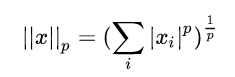（2）常用正则化方法——惩罚项
在目标函数后面添加一个系数的“惩罚项”是正则化的常用方式，为了防止系数过大从而让模型变得复杂。在加了正则化项之后的目标函数为: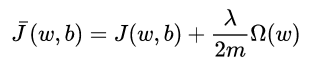正则化时，对应惩罚项为 L1 范数 :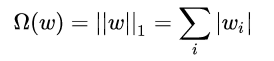正则化时，对应惩罚项为 L2 范数: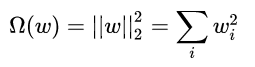从上式可以看出，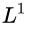正则化通过让原目标函数加上了所有特征系数绝对值的和来实现正则化，而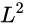正则化通过让原目标函数加上了所有特征系数的平方和来实现正则化。
两者都是通过加上一个和项来限制参数大小，却有不同的效果： 正则化更适用于特征选择，而 正则化更适用于防止模型过拟合。
3、arg
arg max f(x): 当f(x)取最大值时，x的取值：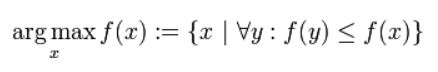arg min f(x)：当f(x)取最小值时，x的取值
表示使目标函数取最小值时的变量值
4、梯度下降
P.S:参考资料http://speech.ee.ntu.edu.tw/~tlkagk/courses_ML17.html
计算过程就是沿梯度下降的方向求解极小值
迭代公式为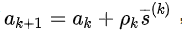,其中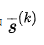代表梯度负方向，表示梯度方向上的搜索步长。梯度方向我们可以通过对函数求导得到。 一般确定步长的方法是由线性搜索算法来确定，即把下一个点的坐标看做是ak+1的函数，然后求满足f(ak+1)的最小值的ak+1即可。
算法迭代的终止条件是梯度向量的幅值接近0即可。
5、线性回归的概率论来源——极大似然估计
（1）贝叶斯分类（公式）
经典贝叶斯公式：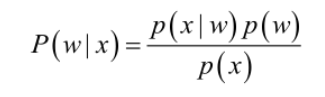在机器学习中的形式变成：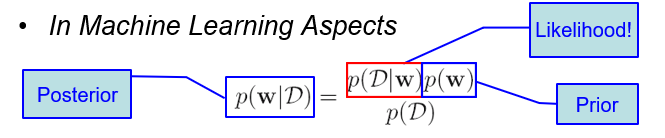（2）似然函数
实际问题中我们能获得的数据可能只有有限的样本数据，而先验概率和类条件概率（各类的总体分布）都是未知的。我们需要对其中的类条件概率进行估计，并将概率密度估计问题转换成参数估计问题 ，由此我们用到极大似然的方法。
条件概率密度函数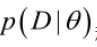称为相对于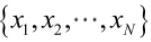的θ的似然函数。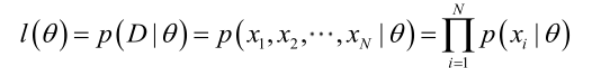因此，极大似然估计的本质就是求解贝叶斯公式中的似然项（类条件概率），也就是机器学习中“利用已知事物特征来输出数据”的过程。
（3）线性模型
接下来由似然函数构建线性模型，假设模型是关于自变量的线性函数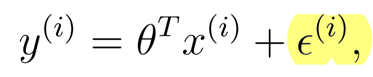假设偏差项符合高斯分布，则有：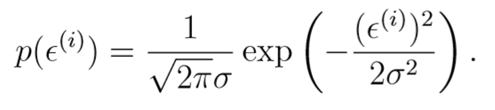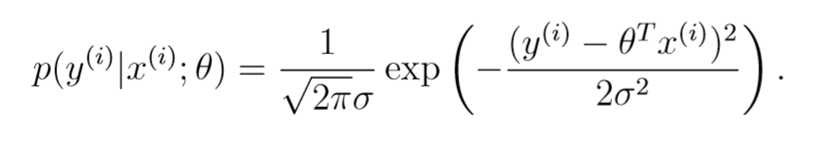那么可得这个线性模型的关于θ的似然函数：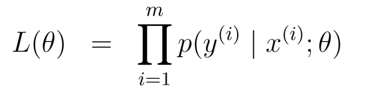或者写成更容易理解的形式：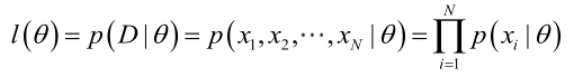进行对数化之后变成：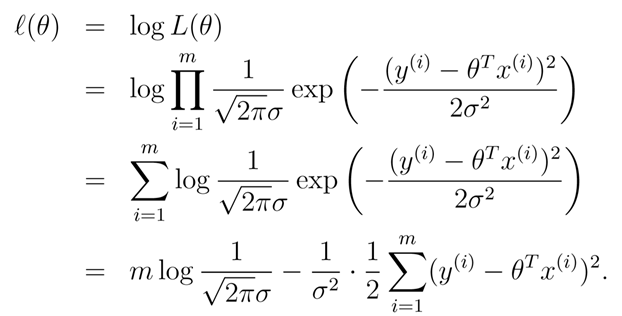由此，我们的问题转换成了求解能够得到极大似然的参数θ的参数估计问题。
我们的目的是令似然函数最大，因此要求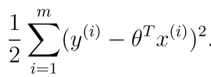这一项取最小，它在形式上等同于前面提到的Loss function，这也就是线性回归的判断标准之一——均方误差。
（4）贝叶斯估计
上面的极大似然估计求参数的方式存在一些显著的问题：
模型的复杂度会被两个因素所控制：基函数的数目（的维数）和样本的数目。尽管为对数极大似然估计加上一个正则项（或者是参数的先验分布），在一定程度上可以限制模型的复杂度，防止过拟合，但单纯使用极大似然估计总是会令模型过于复杂以至于产生过拟合。
于是我们采用贝叶斯回归（最大后验概率估计MAP）的方式来改善极大似然估计中的不足。参数θ是服从一定先验分布的随机变量，则新的数据集D出现后，我们可以用于更新参数θ的估计，更新后的分布就是后验概率分布。
贝叶斯估计的形式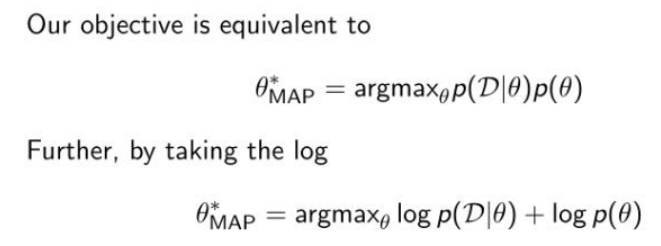从某种意义上，贝叶斯点估计是选取一个随机变量θ的统计值来代替分布，但真正意义上的贝叶斯估计方法应该是使用参数空间中所有的参数分别建立模型，用所有的模型进行估计，取所有估计值的期望为最终估计值，权值根据参数的概率分布计算，并加以降低计算量的方法。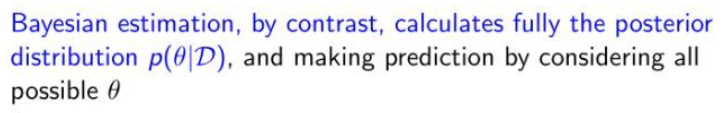之后我们再求这个模型的关于θ（下面用w代替）的似然函数并进行对对数化：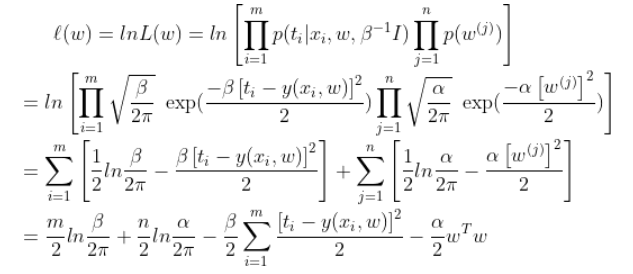求最大的问题可以转换为求后面两项的最小值：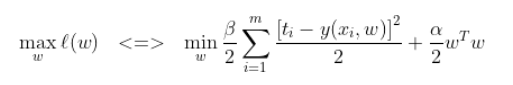也就是求：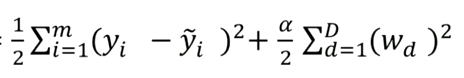的最小值，我们将其定义为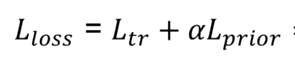也就是在前面讲到的加入正则项后的Loss function，由此整个链条可以完整穿起来，从线性模型->似然函数->极大似然估计下的Loss function->正则化->贝叶斯估计下的Loss function。


展开全文机器学习
• 回归线性 可以方便地在模型上进行自动建模，也可以在variate的基础上使用python并可以在python上使用lenguaje ... 对数线性对数和对数线性对数 co科动物 信息无用信息（VIF） 虚拟变量（变量ficticias） Regr
• 对数可能性：可能性的自然对数 边际模型：一种线性模型，说明对同一主题的重复响应措施。一般线性模型的五个扩展： : 。 稳健的标准误差：一种在异方差下获得OLS系数的无偏标准误差的技术 。 Bonferonni校正：将...
• 4 对数线性语言模型 本节讨论另一种语言模型：对数线性语言模型。... 计算特征：对数线性语言模型围绕特征这个概念。特征是“上下文中某个对预测下一个词有用的东西”。更精确地，我们定义一个特征函

4 对数线性语言模型

本节讨论另一种语言模型：对数线性语言模型。它的计算方法跟上面提到的基于计数的语言模型很不同。

4.1 模型公式化

对数线性语言模型也是计算给定前几个词的条件下下一个词的条件概率，但是方法不一样，基本分为以下几步：

计算特征：对数线性语言模型围绕特征这个概念。特征是“上下文中某个对预测下一个词有用的东西”。更精确地，我们定义一个特征函数ϕ(et−1t−n+1)<!--//--><![CDATA[//><!--
\phi(e_{t-n+1}^{t-1})
//--><!]]>，以上下文为输入，输出一个实值特征向量x∈ℝN<!--//--><![CDATA[//><!--
x \in \mathbb{R}^{N}
//--><!]]>来用N<!--//--><![CDATA[//><!--
N
//--><!]]>个特征描述上下文。
计算得分：有了特征向量之后，我们就要用它预测每个单词的概率。为此，我们计算一个得分向量s∈ℝ|V|<!--//--><![CDATA[//><!--
s \in \mathbb{R}^{|V|}
//--><!]]>对应每个词的似然。我们用模型参数W∈ℝ|V|×N,b∈ℝN<!--//--><![CDATA[//><!--
W \in \mathbb{R}^{|V| \times N},b \in\mathbb{R}^N
//--><!]]>来计算得分向量： s=Wx+b<!--//--><![CDATA[//><!--
\begin{equation}
s = Wx + b
\end{equation}
//--><!]]>
计算概率：把得分向量转换成概率向量： p=softmax(s)<!--//--><![CDATA[//><!--
\begin{equation}
p = softmax(s)
\end{equation}
//--><!]]>

解读：p<!--//--><![CDATA[//><!--
p
//--><!]]>表示了在上下文et−1t−n+1<!--//--><![CDATA[//><!--
e_{t-n+1}^{t-1}
//--><!]]>之后，每个词出现的概率。p<!--//--><![CDATA[//><!--
p
//--><!]]>本身是长度为|V|<!--//--><![CDATA[//><!--
|V|
//--><!]]>的向量，向量里的值表示相应位置的词出现的概率。每个词都有一个index，这个index跟p<!--//--><![CDATA[//><!--
p
//--><!]]>的index相对应。

4.2 学习模型参数

首先我们要定义损失函数l<!--//--><![CDATA[//><!--
l
//--><!]]>，一个表达我们在训练数据上做的有多差的函数。大多数情况下，我们假设这个损失等于负对数似然： l(εtest;θ)=−logP(εtest⎪θ)=−∑E∈εtrainlogP(E⎪θ)<!--//--><![CDATA[//><!--
\begin{equation}
l(\varepsilon_{test};\theta) = -log P(\varepsilon_{test} \bracevert \theta) = - \sum_{E \in \varepsilon_{train}} logP(E \bracevert \theta)
\end{equation}
//--><!]]>

解读：这里的参数不再是P(et⎪et−1t−n+1)<!--//--><![CDATA[//><!--
P(e_{t} \bracevert e_{t-n+1}^{t-1})
//--><!]]>，而是W,b<!--//--><![CDATA[//><!--
W,b
//--><!]]>。不同的参数得到不同的损失，我们要通过训练找到那个使损失最小的参数。比如，我们要计算一个句子E<!--//--><![CDATA[//><!--
E
//--><!]]>的损失，就计算参数对于E<!--//--><![CDATA[//><!--
E
//--><!]]>的负对数似然。通过之前的 计算概率 公式依次计算E<!--//--><![CDATA[//><!--
E
//--><!]]>的分句的条件概率，再用链式法则计算参数对于E<!--//--><![CDATA[//><!--
E
//--><!]]>的似然P(E⎪θ)<!--//--><![CDATA[//><!--
P(E \bracevert \theta)
//--><!]]>，相应也得到了负对数似然。

用随机梯度下降更新参数。为了要保证训练过程稳定，还有其他东西要考虑：

调整学习率：一开始学习率比较大，然后逐渐减少。
早停：通常会留出一个开发集(验证集)，在这个集上测量对数似然，然后保留那个最大对似然的模型。这是为了防止过拟合。另一个防止过拟合的办法是当开发集上的对数似然停止提高的时候减小学习率。
洗牌顺序：有的时候顺序有所偏向，为了防止最后训练的模型更贴合结尾部分的数据，我们需要把整个数据训练的顺序洗牌。
4.3 对数线性模型的导数

略

4.4 语言模型的其他特征

对数线性模型好的原因是它允许我们灵活地选择我们认为对预测下一个词有用的特征，包括：

上下文特征：如之前所说。
上下文类：把相似的词归为一类。
词后缀特征：比如ing。
词袋特征： 跟只用句子里前n个词相反，我们可以用之前所有的词，然后不顾顺序。这样我们会损失排列信息，但是会知道哪些词会一同出现。
5 神经网络和前馈语言模型

略

6 循环神经网络语言模型

6.1 - 6.4 略

6.5 Online, Batch, and Minibatch Training

对于每一个样例进行参数更新的学习叫做online学习。与之相反，batch学习将整个训练集视为单个单元，计算这个单元的梯度，然后在遍历所有数据之后进行参数更新。

这两个更新策略各有权衡：

online学习更快地找到一个好的答案。
训练结束后，batch学习更稳定，因为它不受最后看见的数据的影响。
batch学习更容易陷入局部最优解。online学习的随机性使得模型能够跳出局部最优，找到一个全局最优。
minibatching是以上两者的折中。minibatching每次计算n个训练样例的梯度。

展开全文• （1）基本概念 图像的点运算包含线性变换和非线性变换，其中,非线性变换分为对数变换、幂次变换、指数变换等。这几种变换都能有效的改变图像的亮度和对比度，然而，其中的参数却需要人为设定,设定的好,才能得到效果...

（1）基本概念
图像的点运算包含线性变换和非线性变换，其中,非线性变换分为对数变换、幂次变换、指数变换等。这几种变换都能有效的改变图像的亮度和对比度，然而，其中的参数却需要人为设定,设定的好,才能得到效果。那么问题来了，是否存在一种变换，不需要人为设定参数，就可以改善图像的对比度？
显然，从图像对比度的定义出发（简单来说，对比度就是最黑与最白亮度单位的相除值），如果一幅图像占据的灰度级范围更大，且每一像素的灰度级分布均匀，则该图像就具有更高的对比度，更丰富的细节，以及更大的信息量。那么，现在的任务，就是找到一个变换函数s=T(r),使得灰度级r经过映射后得到的灰度级s，呈均匀分布。
上一篇博文中强调过，像素的灰度级r可以看作是一个随机变量（没错，就是随机变量，曾经以为这辈子除了考试再不会用到的概率论知识，竟然这么快就见面了），则灰度级的分布就可以用随机变量的累计分布函数和累计概率密度函数来刻画和描述。捋一捋现在的条件，不难发现，像素原灰度级r已知，r的概率密度函数P(r)已知，变换后灰度级s的概率分布P(s)也已知（因为期望得到的灰度级s呈均匀分布，假设像素最高灰度级为L，则P(s)=1/(L-1)）。很简单，这就相当于概率论中的一个题型“求一维随机变量函数的分布问题，即已知X的概率分布，求Y=g(X)的概率分布”。遇到这种问题，我就记着老师讲的“什么都不要管，先把F(y)=P{Y<y}=P{g(X)<y}写上去，两分到手了”，可是，很快我发现推不下去了。翻翻书，《数字图像处理_原理与实践》的作者在这里是直接“由概率理论可以得到如下公式…”，我不是作者，没有他那样扎实的数学功底，如果我也一带而过，那不叫懂，而是不懂装懂。
于是查阅各种资料，终于发现,我漏了一个很重要的条件:为了使变换后的灰度级从白到黑的次序不变，变换函数T(r)应该满足单调递增的条件（仔细想想确是这样）。从下面的图上看更明显,s和r是一一对应的关系,即S<s就等价于R<r,有了这一步，就能继续推导：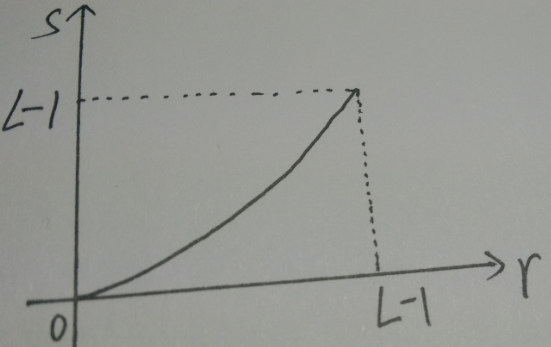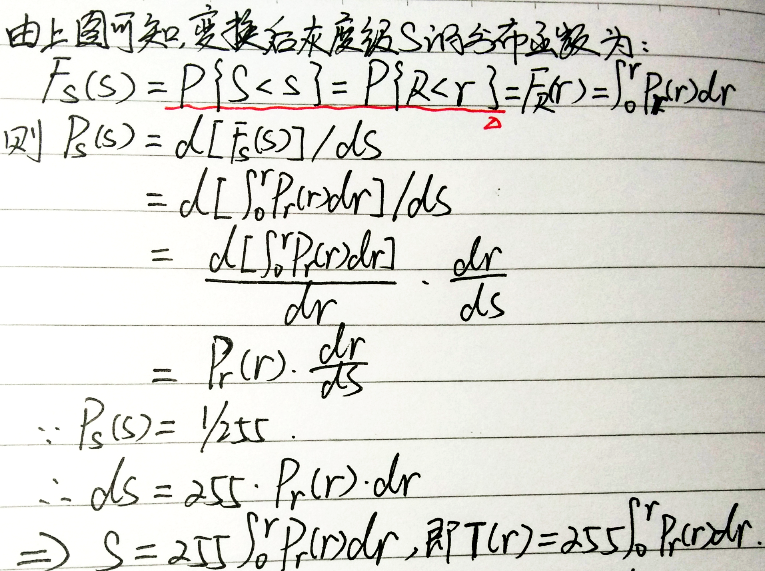然而，上述推导方法是以灰度级r为连续随记变量为基础讨论的,当灰度级r为离散值时,显然可用如下公式表示: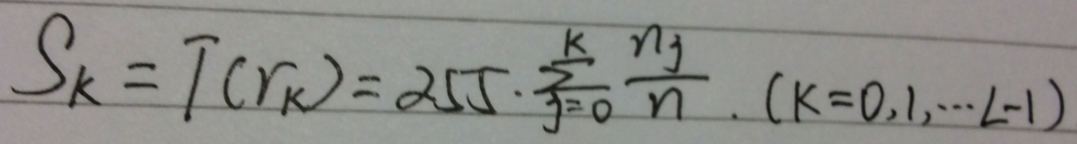至此为止，推导结束（第一次感觉数学这么有用,哈哈）。

（2）编码实现
MATLAB中图像处理工具箱提供了用以实现灰度均衡算法的函数histeq(),语法形式为：
[J,T]=histeq(I,n);
J=histeq(I,n)   ;其中，I表示原始图像，J是灰度均衡化后的输出图像，T是变换矩阵（即返回能将图像I的直方图变换成图像J的直方图的变换T）。参数n指定直方图均衡化后的灰度级数，默认值为64。

下面是自己根据上面数学推导的结果，分步骤完成的灰度均衡化算法代码的实现。
% ***************************Copyright 2016[c]**************************
% ************************Declaration************************************
% File name:        histogram_equalization
% Author:           靖harry
% Date:             16-Jul-2016 11:07:03
% Version Number:   1.0
% Abstract:
%   Enhance the contrast of images by transforming the values in an
%   intensity image, so that the histogram of the output image
%   approximately equalized  histogram.
% *********************************end*********************************
clear
clc
I1=rgb2gray(I1);
[m,n]=size(I1);
L=256;  % total gray_level

%count the number of gray_level pixels respectively.%
nk=zeros(1,256);
for i1=1:m
for j1=1:n
nk(I1(i1,j1)+1)=nk(I1(i1,j1)+1) + 1 ;
end
end

pk=nk/(m*n);  % calculate the PDF of original gray_level.

%figure out the values of transformed gray_level.%
sk=zeros(1,256);
for i2=1:L
for j2=1:i2
sk(i2)=sk(i2)+pk(j2);
end
end
sk=fix((L-1)*sk);  % take integer part of variabel sk.
%确定映射关系，得到新图像I2.%
I2=I1;
for i3=1:m
for j3=1:n
I2(i3,j3)=sk(I1(i3,j3)+1);
end
end

I3=histeq(I1,256);%用matlab自带函数实现均匀化得到的图像
subplot(3,2,1),imshow(I1),title('original image');
subplot(3,2,2),imhist(I1),title('original histogram');
subplot(3,2,3),imshow(I2),title('transformed image by myself');
subplot(3,2,4),imhist(I2),title('transformed histogram by myself');
subplot(3,2,5),imshow(I3),title('transformed image by matlab');
subplot(3,2,6),imhist(I3),title('transformed histogram by matlab');
以下是分别用几张图像实验的效果: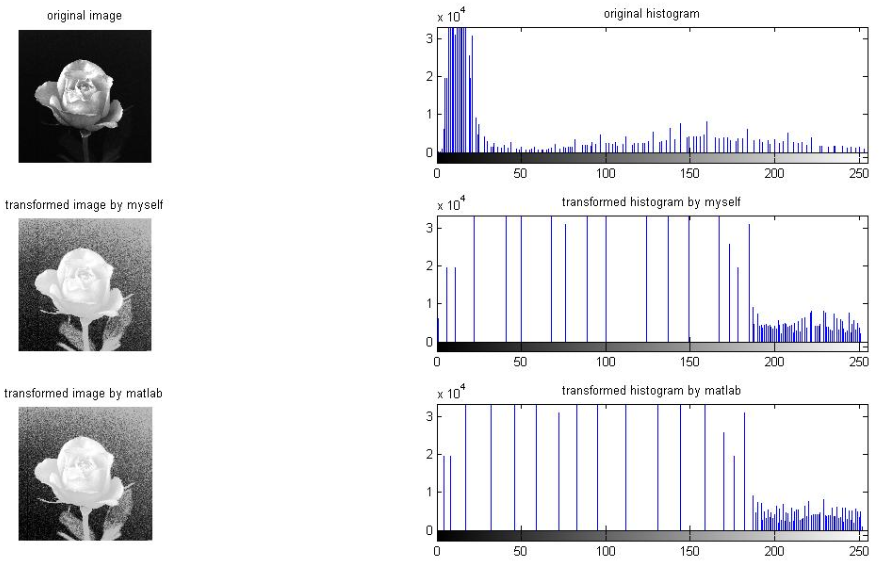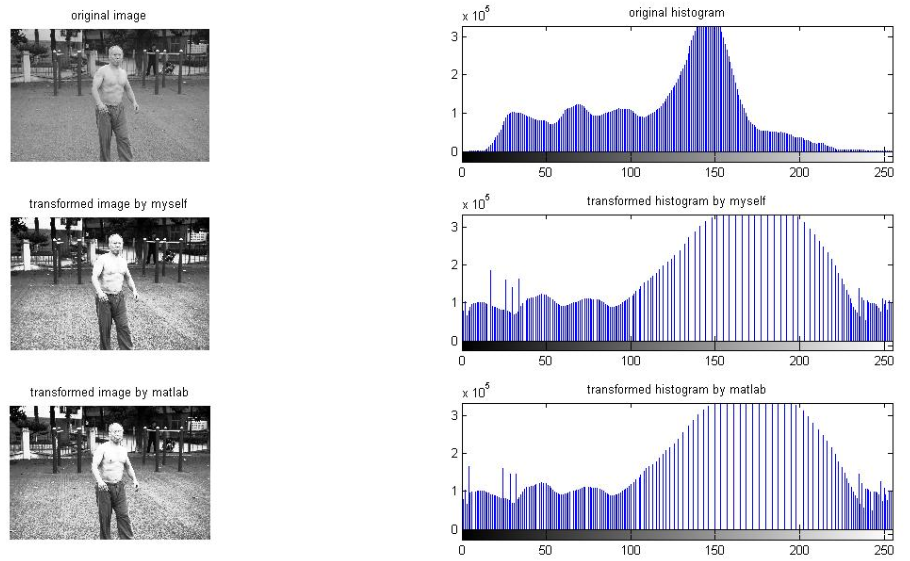再来一张: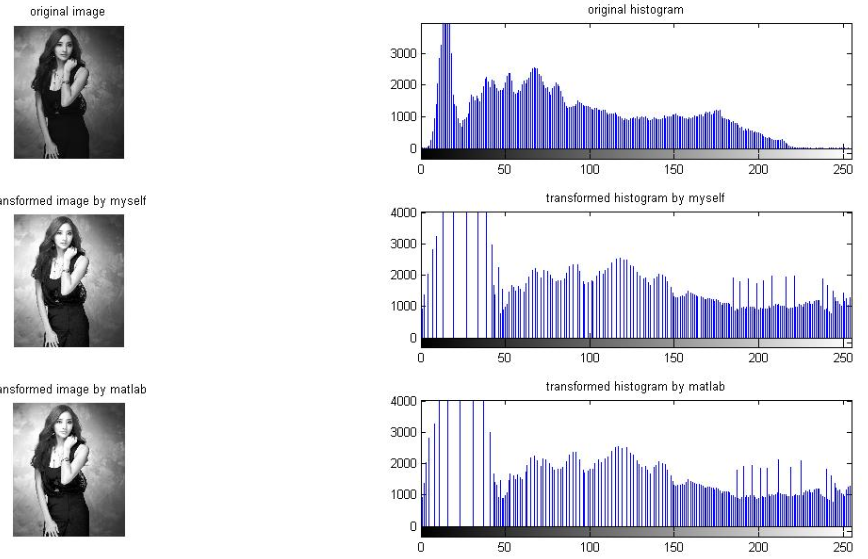展开全文• 一个事件发生的几率（odds）为该事件发生的概率与不发生概率的比值，几率的取值范围为[0,+∞)，其对数的取值范围为实数域，所以，可以将对数几率作为因变量构建线性回归模型： 由此可得，即P...
• 概念例子2TF-IDF 的4个变种变种1：通过对数函数避免 TF 线性增长用 Log，也就是对数函数，对 TF 进行变换，就是一个不让 TF 线性增长的技巧。变种2：标准解决长文档、短文档问题**变种3：对数函数处理 IDF实例1笔...
• 为了说明广义线性模型，我们先需要知道指数族的概念，因为广义线性模型是指数族的延伸，首先我们给出指数族的一般形式： 这里的????被称为分布的特性参数（也被称为标准参数或范参数），其中的T(y)被称为充分统计量...机器学习
• 目录1.基本概念2.可调参数3.调参技巧3.1.Random Search...权重初始4.7.Batch Normalization批量归一5.泛化5.1.欠拟合5.2.过拟合Dropout层：λ\lambdaλ调参参考 1.基本概念 超参数： 如学习率ααα、adam方法的β神经网络 算法 机器学习 人工智能
• 19.6非线性微分方程组的线性化 第20章　最优控制理论 20.1最优控制的特性 20.2其他终止条件 20.3自治问题 20.4经济应用 20.5无限时间跨度 20.6动态分析的局限性 附录I　希腊字母 附录Ⅱ　数学符号 附录Ⅲ　主要参考...
• 逻辑回归是线性模型，其中sigmoid函数只是非线性激活函数 极大似然视角下的Logistic 极大似然与伯努利分布 假设 x,y∼B(±1,p)x,y∼B(±1,p) 考虑一个二分类问题：f(x)→{+1,−1}f(x)→{+1,−1} ： 其极大条件似然...
• 找出我们的数据是否偏斜，以及如何通过不同的变换（Box-Cox，Tukey，对数，立方/平方根变换，数据归一/标准）解决偏斜问题 有效线性回归的假设，探索性数据分析的描述性统计量等我们尝试了以下机器学习算法： ...HTML
• 10.1.4对数频率稳定判据 10.1.5开环频域性能指标 10.2Bode图 10.3Nyquist图 10.4系统稳定性的判定 10.4.1用Bode图法判断系统稳定性 10.4.2用Nyquist曲线法判断系统稳定性 第11章基于频域滤波的图像分析与实现...
• 日本东北大学博士，四川大学电气工程学院教授、自动系系主任，四川省信息与自动技术重点实验室主任。主要专注于先进控制理论与人工智能算法研究、嵌入式计算与实时智能系统的研究与开发、机器人与智能装备的智能...
• 　3.2.2 对数和对比度拉伸变换 　3.2.3 亮度变换的一些实用M函数 　3.3 直方图处理与函数绘图 　3.3.1 生成并绘制图像的直方图 　3.3.2 直方图均衡 　3.3.3 直方图匹配（规定） 　3.4 空间滤波 　3.4.1 线性空间...
• 函数概念的演变 极限思想的历史发展 对数理论的创立 微积分的诞生 函数的连续性 微分中值定理 分析严格 变分法的诞生 复数 复变函数论的创立 实变函数论 泛函分析 函数逼近论 傅立叶分析 非标准分析...
• 图像的灰度非线性变换之对数变换、伽马变换 [Python图像处理] 十七.图像锐化与边缘检测之Roberts算子、Prewitt算子、Sobel算子和Laplacian算子 [Python图像处理] 十八.图像锐化与边缘检测之Scharr算子、Canny算子...
• 　第２节　定性资料的对数线性模型分析 　第３节　定性资料的logistic回归分析 　第４篇　试验设计与回归分析 第１章　回归分析的种类与简单回归分析 　第１节　回归分析的任务和种类 　第２节　直线回归与相关...
• 45 3.4.5 Pólya计数定理 47 3.5 博弈论 50 3.5.1 博弈树 50 3.5.2 SG函数 51 3.5.3 Nim游戏与Nim和 53 3.6 数论 54 3.6.1 整除 54 3.6.2 不定方程 57 3.6.3 同余方程与欧拉定理 58 3.6.4 原根、离散对数和二项同余 ...
• 6．10．4 极分集 6．10．5 频率分集 6．10．6 时间分集 6．11 RAKE接收机 6．12 交织 6．13 信道编码原理 6．14 分组码 6．14．1 分组码举例 6．14．2 Reed—Solomon码的实例研究 6．15 卷积码 6．15．1...无线通信原理
• ## 无线通信原理与应用第二版中文版

千次下载 热门讨论 2010-10-31 20:52:41
6．10．4 极分集 6．10．5 频率分集 6．10．6 时间分集 6．11 RAKE接收机 6．12 交织 6．13 信道编码原理 6．14 分组码 6．14．1 分组码举例 6．14．2 Reed—Solomon码的实例研究 6．15 卷积码 6．15．1...
• 2.17.3 决策树学习基本算法 2.17.4 决策树算法优缺点 2.17.5熵的概念以及理理解 2.17.6 信息增益的理理解 2.17.7 剪枝处理理的作⽤用及策略略? 2.18 ⽀支持向量量机 2.18.1 什什么是⽀支持向量量机 2.18.2 ⽀支持...